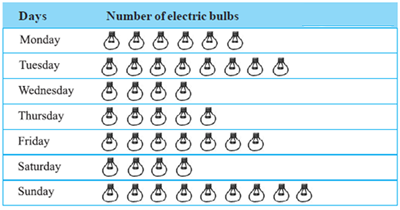Mathematics
Easy

Question

# The number of electric bulbs sold on Wednesday is ______.## 2478Hint:

## The correct answer is: 4

###Here we have to find the number of bulb sold in Wednesday,Firstly, In fig, we given by each days and also the number of bulbs,On Wednesday , see on table then,Count the total number of bulbs Therefore, the total number of bulb sold in Wednesday is 4.The correct answer is 4 (option (b)).

In this question , We have given by table we just find out the given day and count the number of bulb in the row.

### Related Questions to study#### With Turito Foundation.#### Get an Expert Advice From Turito.